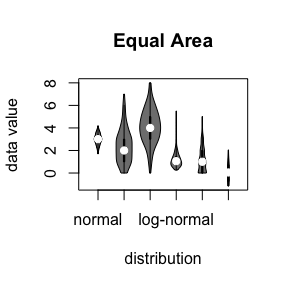# Controlling Violin Plot Area

#### 2020-06-15

While boxplots have become the de facto standard for plotting the distribution of data this is a vast oversimplification and may not show everything needed to evaluate the variation of data. This is particularly important for datasets which do not form a Gaussian “Normal” distribution that most researchers have become accustomed to.

While density plots are helpful in this regard, they can be less aesthetically pleasing than boxplots and harder to interpret for those familiar with boxplots. Often the only ways to compare multiple data types with density use slices of the data with faceting the plotting panes or overlaying density curves with colours and a legend. This approach is jarring for new users and leads to cluttered plots difficult to present to a wider audience.

##Violin Plots

Therefore violin plots are a powerful tool to assist researchers to visualise data, particularly in the quality checking and exploratory parts of an analysis. Violin plots have many benefits:

• Greater flexibility for plotting variation than boxplots
• More familiarity to boxplot users than density plots
• Easier to directly compare data types than existing plots

As shown below for the iris dataset, violin plots show distribution information that the boxplot is unable to.

library("vioplot")
## Loading required package: sm
## Package 'sm', version 2.2-5.6: type help(sm) for summary information
## Loading required package: zoo
##
## Attaching package: 'zoo'
## The following objects are masked from 'package:base':
##
##     as.Date, as.Date.numeric
data(iris)
boxplot(iris$Sepal.Length[iris$Species=="setosa"], iris$Sepal.Length[iris$Species=="versicolor"], iris$Sepal.Length[iris$Species=="virginica"], names=c("setosa", "versicolor", "virginica"))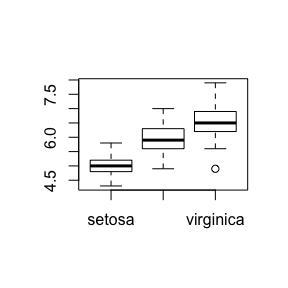vioplot(iris$Sepal.Length[iris$Species=="setosa"], iris$Sepal.Length[iris$Species=="versicolor"], iris$Sepal.Length[iris$Species=="virginica"], names=c("setosa", "versicolor", "virginica"))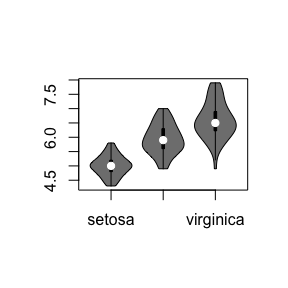##Violin Plot Area

However there are concerns that existing violin plot packages (such as ) scales the data to the most aesthetically suitable width rather than maintaining proportions comparable across data sets. Consider the differing distributions shown below:

par(mfrow=c(3, 1))
par(mar=rep(2, 4))
plot(density(iris$Sepal.Length[iris$Species=="setosa"]), main="Sepal Length: setosa", col="green")
plot(density(iris$Sepal.Length[iris$Species=="versicolor"]), main="Sepal Length: versicolor", col="blue")
plot(density(iris$Sepal.Length[iris$Species=="virginica"]), main="Sepal Length: virginica", col="palevioletred4")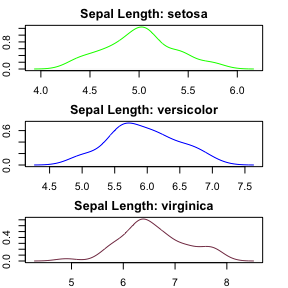par(mfrow=c(1, 1))

#Comparing datasets

Neither of these plots above show the relative distribtions on the same scale, even if we match the x-axis of a density plot the relative heights are obscured and difficult to compare.

par(mfrow=c(3, 1))
par(mar=rep(2, 4))
xaxis <- c(3, 9)
yaxis <- c(0, 1.25)
plot(density(iris$Sepal.Length[iris$Species=="setosa"]), main="Sepal Length: setosa", col="green", xlim=xaxis, ylim=yaxis)
plot(density(iris$Sepal.Length[iris$Species=="versicolor"]), main="Sepal Length: versicolor", col="blue", xlim=xaxis, ylim=yaxis)
plot(density(iris$Sepal.Length[iris$Species=="virginica"]), main="Sepal Length: virginica", col="palevioletred4", xlim=xaxis, ylim=yaxis)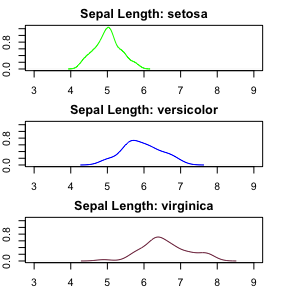par(mfrow=c(1, 1))

This can somewhat be addressed by overlaying density plots:

par(mfrow=c(1, 1))
xaxis <- c(3, 9)
yaxis <- c(0, 1.25)
plot(density(iris$Sepal.Length[iris$Species=="setosa"]), main="Sepal Length", col="green", xlim=xaxis, ylim=yaxis)
lines(density(iris$Sepal.Length[iris$Species=="versicolor"]), col="blue")
lines(density(iris$Sepal.Length[iris$Species=="virginica"]), col="palevioletred4")
legend("topright", fill=c("green", "blue", "palevioletred4"), legend=levels(iris$Species), cex=0.5)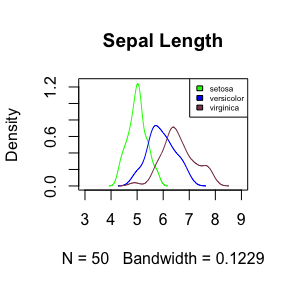This has the benefit of highlighting the different distributions of the data subsets. However, notice here that a figure legend become necessary, plot axis limits need to be defined to display the range of all distribution curves, and the plot quickly becomes cluttered if the number of factors to be compared becomes much larger. ##Area control in Violin plot Therefore the areaEqual parameter has been added to customise the violin plot to serve a similar purpose: vioplot(iris$Sepal.Length[iris$Species=="setosa"], iris$Sepal.Length[iris$Species=="versicolor"], iris$Sepal.Length[iris$Species=="virginica"], names=c("setosa", "versicolor", "virginica"), main="Sepal Length", areaEqual = T)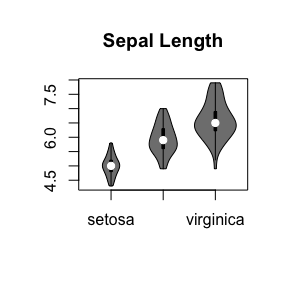If we compare this to the original vioplot functionality (defaulting to areaEqual = FALSE) the differences between the two are clear. par(mfrow=c(2,1)) par(mar=rep(2, 4)) vioplot(iris$Sepal.Length[iris$Species=="setosa"], iris$Sepal.Length[iris$Species=="versicolor"], iris$Sepal.Length[iris$Species=="virginica"], names=c("setosa", "versicolor", "virginica"), main="Sepal Length (Equal Width)", areaEqual = F) vioplot(iris$Sepal.Length[iris$Species=="setosa"], iris$Sepal.Length[iris$Species=="versicolor"], iris$Sepal.Length[iris$Species=="virginica"], names=c("setosa", "versicolor", "virginica"), main="Sepal Length (Equal Area)", areaEqual = T)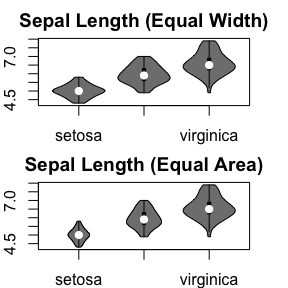par(mfrow=c(1,1)) Note that areaEqual is considering the full area of the density distribution before removing the outlier tails. We leave it up to the users discretion which they elect to use. The areaEqual functionality is compatible with all of the customisation used in discussed in the main vioplot vignette vioplot(iris$Sepal.Length[iris$Species=="setosa"], iris$Sepal.Length[iris$Species=="versicolor"], iris$Sepal.Length[iris$Species=="virginica"], names=c("setosa", "versicolor", "virginica"), main="Sepal Length (Equal Area)", areaEqual = T, col=c("lightgreen", "lightblue", "palevioletred"), rectCol=c("green", "blue", "palevioletred3"), lineCol=c("darkolivegreen", "royalblue", "violetred4"), border=c("darkolivegreen4", "royalblue4", "violetred4"))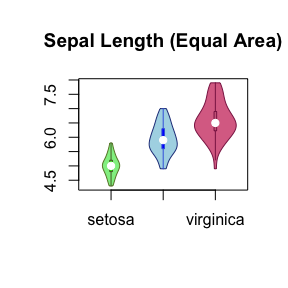The violin width can further be scaled with wex, which maintains the proportions across the datasets if areaEqual = TRUE: vioplot(iris$Sepal.Length[iris$Species=="setosa"], iris$Sepal.Length[iris$Species=="versicolor"], iris$Sepal.Length[iris\$Species=="virginica"], names=c("setosa", "versicolor", "virginica"), main="Sepal Length (Equal Area)", areaEqual = T, col=c("lightgreen", "lightblue", "palevioletred"), rectCol=c("green", "blue", "palevioletred3"), lineCol=c("darkolivegreen", "royalblue", "violetred4"), border=c("darkolivegreen4", "royalblue4", "violetred4"), wex=1.25)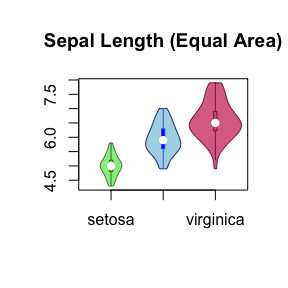## Comparing distributions

Notice the utility of areaEqual for cases where different datasets have different underlying distributions:

vioplot(rnorm(200, 3, 0.5), rpois(200, 2.5),  rbinom(100, 10, 0.4), rlnorm(200, 0, 0.5), rnbinom(200, 10, 0.9), rlogis(20, 0, 0.5), areaEqual = F, main="Equal Width", xlab="distribution", ylab="data value", names=c("normal", "poisson", "binomial", "log-normal", "neg-binomial", "logistic"))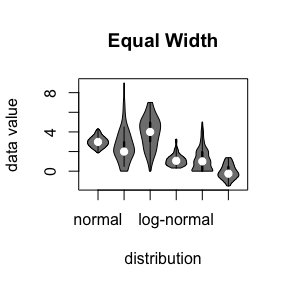vioplot(rnorm(200, 3, 0.5), rpois(200, 2.5),  rbinom(100, 10, 0.4), rlnorm(200, 0, 0.5), rnbinom(200, 10, 0.9), rlogis(20, 0, 0.5), areaEqual = T, main="Equal Area", xlab="distribution", ylab="data value", names=c("normal", "poisson", "binomial", "log-normal", "neg-binomial", "logistic"))